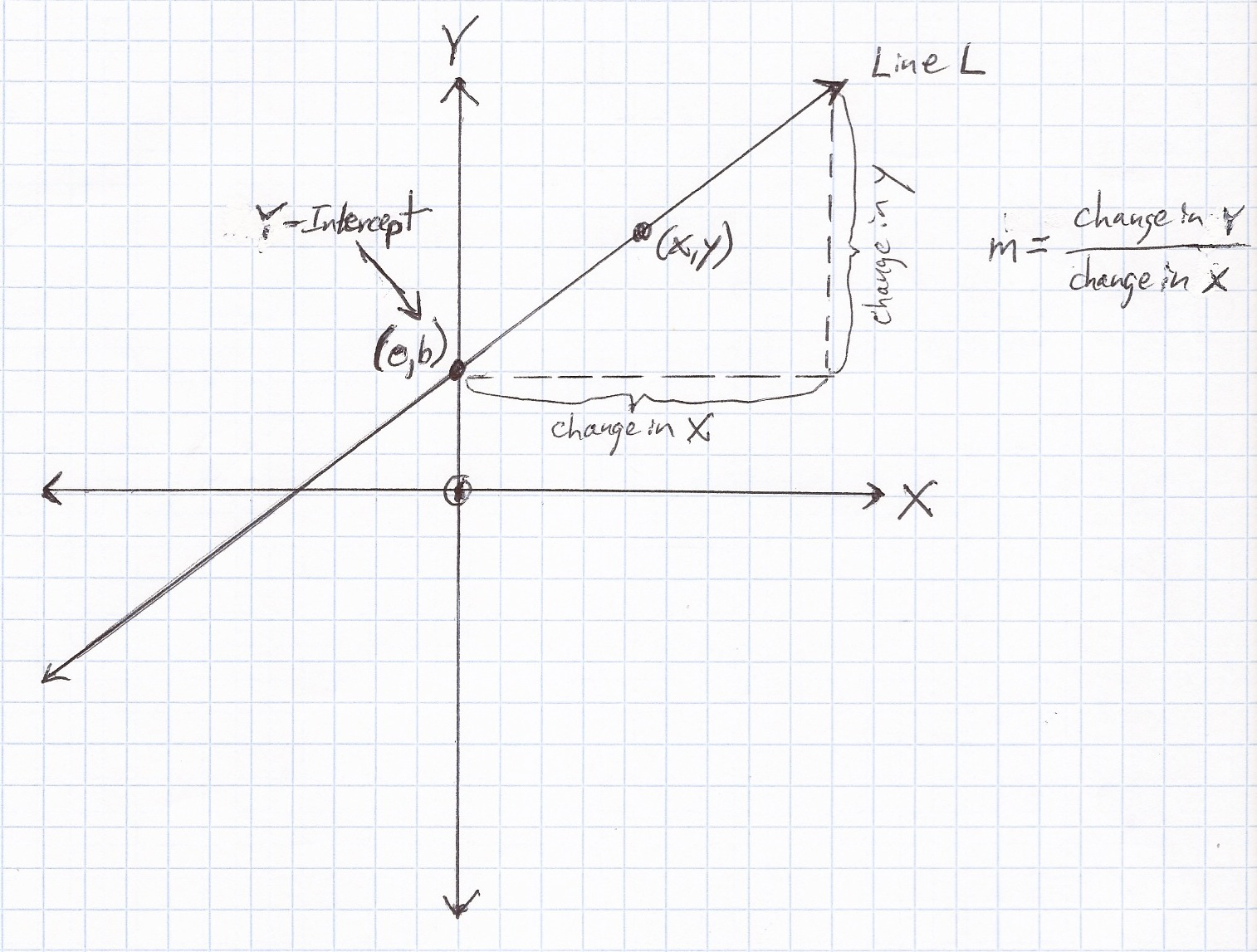## Line

Line = Flat Straight Figure Extending Infinitely Through Two PointsBasicsA line is a flat straight figure extending infinitely through two points.  (See Line L above.)
x-axis = horizontal
y-axis = vertical
x = horizontal coordinate
y = vertical coordinate
(x, y) = point representing horizontal and vertical coordinates on Line L
(o, b) = y-intercept = point where Line L crosses the y-axis
m = slope

Formulas (provided by Mathematics Formula Sheet)
slope of a line$\bf\displaystyle m=\frac{{{y}_{2}}-{{y}_{1}}}{{{x}_{2}}-{{x}_{1}}}$

slope-intercept form of the equation of a line$\bf\displaystyle y=mx+b$

point-slope form of the equation of a line$\bf\displaystyle y-{{y}_{1}}=m(x-{{x}_{1}})$

Formulas (NOT provided by Mathematics Formula Sheet)
slope as change$\bf\displaystyle m=\frac{{{change\;in\;y}}}{{{change\;in\;x}}}$

slope-intercept b$\bf\displaystyle b=y-mx$

standard form of the equation of a line$\bf\displaystyle ax+by+c=0$